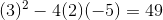# High School Math : Understanding the Discriminant

## Example Questions

### Example Question #1 : Understanding The Discriminant

Use the discriminant to determine the nature of the roots: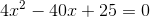imaginary rootsrational rootsirrational rootsrational rootimaginary rootirrational roots

Explanation:

The formula for the discriminant is: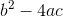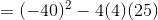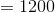Since the discriminant is positive and not a perfect square, there areirrational roots.

### Example Question #2 : Understanding The Discriminant

Use the discriminant to determine the nature of the roots: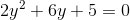irrational rootsrational rootsimaginary rootrational rootimaginary rootsimaginary roots

Explanation:

The formula for the discriminant is: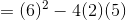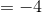Since the discriminant is negative, there areimaginary roots.

### Example Question #3 : Understanding The Discriminant

Use the discriminant to determine the nature of the roots: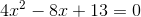imaginary rootreal roots

Cannot be determinedimaginary rootsreal rootimaginary roots

Explanation:

The formula for the discriminant is: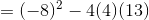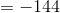Since the discriminant is negative, there areimaginary roots.

### Example Question #4 : Understanding The Discriminant

Given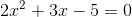, what is the value of the discriminant?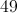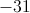In general, the discriminant is.
In this particual case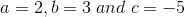.
Plug in these three values and simplify: